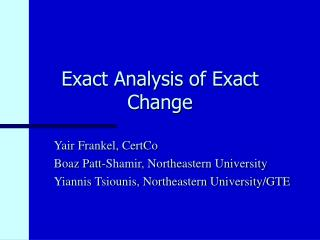DownloadDownload PresentationExact Analysis of Exact Change

# Exact Analysis of Exact Change

Télécharger la présentation## Exact Analysis of Exact Change

- - - - - - - - - - - - - - - - - - - - - - - - - - - E N D - - - - - - - - - - - - - - - - - - - - - - - - - - -
##### Presentation Transcript

1. Exact Analysis of Exact Change Yair Frankel, CertCo Boaz Patt-Shamir, Northeastern University Yiannis Tsiounis, Northeastern University/GTE

2. At the bank... • YOU: I would like to withdraw N shekels… • THE TELLER: How would you like to have it? • YOU: hmm… I need to make a few exact payments... Northeastern University/ GTE Laboratories

3. Easy cases • No restrictions: up toNpayments (the N-payment problem) • N1-coins are necessary and sufficient • For N = 2m-1: allocate coins 1, 2, 4, 8,..., 2m-1 • pay by the binary representation of P • A single payment of any value P  N (the 1-payment problem) Northeastern University/ GTE Laboratories

4. The k-payment problem Withdraw budget N to enable karbitrary payments with minimal number of coins We need algorithms for: • Coin allocation • Coin dispensing Northeastern University/ GTE Laboratories

5. Applications • Physical cash • Minimize coins in physical withdrawals • “Exact change” ATM machines • Electronic cash: smart cards have limited storage, electronic coins are long sequences... • Construct e-cash systems allowing exact payments • Other resource sharing scenarios Northeastern University/ GTE Laboratories

6. A simple solution • Replicatethe 1-payment solution ktimes : • Split budget into k portions of size N/kand solve for 1-payment for each portion • Requires about klog2(N/k) coins However... • How about payments larger than N/k? • What if N/k is not of the form 2m-1? • Is this the best solution? Northeastern University/ GTE Laboratories

7. Our results • Main result: The optimal solution has about kln(N/k) coins for anyN, k • Result extends to any set of allowed denominations (With greedy dispensing, the “binary” solution works for payments N/k) Northeastern University/ GTE Laboratories

8. This talk... • Some intuition for the lower bound • Description of the coin allocation algorithm • Idea for restricted denominations Northeastern University/ GTE Laboratories

9. The lower bound • Idea: the difficult cases are those where payments are equal • For k payments of 1: need k 1-coins • For k payments of 2: need additional k/2 2-coins... • In general: need k/i coins of value i, therefore total #coins is at least k·(1++ +  +k/N) = k·HN/k k ·ln(N/k) Northeastern University/ GTE Laboratories

10. The characterization Theorem A set of coins solves thek-payment problem if and only if for alli  N/k, the sum of coins of denomination ior less is at leastik. Northeastern University/ GTE Laboratories

11. k k 2 k 3 k 4 N k The allocation algorithm Starting from denomination 1, add the minimal number of coins so that the sum allocated with coins of denominations iis at least ki. Northeastern University/ GTE Laboratories

12. Exact analysis • Optimality: There is no way to allocate less coins for any N, k. • Upper bound: The number of coins allocated by the algorithm is never more than (k+1)HN/(k+1)(k+1) ln(N/(k+1)) (reduction, anyone?) Northeastern University/ GTE Laboratories

13. Restricted denominations: the problem • Not all denominations are available • Related problems: • Change making: dispense a single payment with least number of coins • postage stamp problem Northeastern University/ GTE Laboratories

14. Restricted denominations: the solution • Same basic idea: • Allocate coins while preserving the invariant • Use change making algorithm to allocate the remainder (dynamic programming always works) • Proof is more involved • No specific bounds on the number of coins Northeastern University/ GTE Laboratories